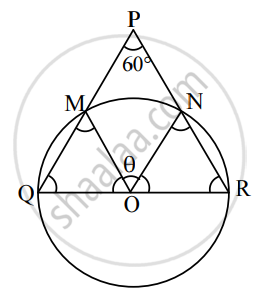# Answer the following : ΔPQR is an equilateral triangle with side 18 cm. A circle is drawn on segment QR as diameter. Find the length of the arc of this circle within the triangle. - Mathematics and Statistics

Sum

ΔPQR is an equilateral triangle with side 18 cm. A circle is drawn on segment QR as diameter. Find the length of the arc of this circle within the triangle.

#### SolutionLet O be the centre of the circle drawn on QR as a diameter.

Let the circle intersect seg PQ and seg PR at points M and N respectively.

Since, l("OQ") = l("OM"),

∴ m∠OMQ = m∠OQM = 60°

∴ m∠MOQ = 60°

Similarly, m∠NOR = 60°

Given, QR = 18 cm

∴ r = 9 cm

∴ θ = 60° = (60 xx pi/180)^"c" = pi^"c"/3

∴ length of the arc MN = S = rθ

= 9 xx pi/3

= 3π cm

Concept: Length of an Arc of a Circle
Is there an error in this question or solution?

#### APPEARS IN

Balbharati Mathematics and Statistics 1 (Arts and Science) 11th Standard Maharashtra State Board
Chapter 1 Angle and its Measurement
Miscellaneous Exercise 1 | Q 2. II (03) | Page 12